This is an automatically generated documentation by LaTeX2HTML utility. In case of any issue, please, contact us at info@cfdsupport.com.

## Baldwin - Lomax algebraic model

The Baldwin-Lomax model (Baldwin and Lomax (1978), ) is a two-layer algebraic which gives the eddy viscosity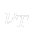as a function of the local boundary layer velocity profile. The model is suitable for high-speed flows with thin attached boundary-layers, typically present in aerospace and turbomachinery applications. It is commonly used in quick design iterations where robustness is more important than capturing all details of the flow physics. The Baldwin-Lomax model is not suitable for cases with large separated regions and significant curvature/rotation effects.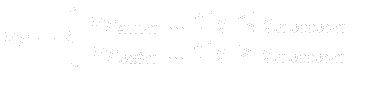(27.17)
where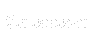is the smallest distance from the surface where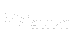is equal to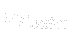: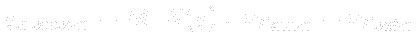(27.18)
The inner region is given by Prandtl – Van Driest formula:(27.19)
where: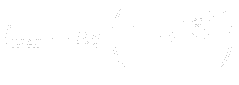(27.20)
and(27.21)
and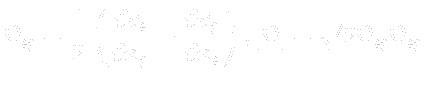(27.22)
The outer region is given by:(27.23)
where:(27.24)
where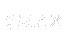and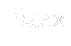are determined from the maximum of the function: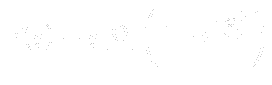(27.25)
where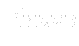is the intermittency factor given by:(27.26)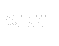is the difference between maximum and minimum speed in the profile. For boundary layers the minimum is always set to zero.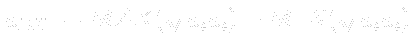(27.27)
The table below gives the model constants present in the formulas above.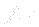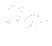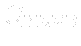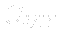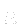K 26.0 1.6 0.3 0.25 0.41 0.0168

Subsections